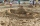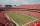# Shoes

Peter decided to measure shoes size of his three friends. Measured accurately and wrote as follows:

Venda and Jenda together 52 cm
Jenda and Zdenda together 58 cm
Zdenda and Venda together 54 cm
a) How long measures Vendy, Jenda and Zdenda shoes together?
b) Determine the length of each shoe individually

Result

s =  82 cm
v =  24 cm
j =  28 cm
z =  30 cm

#### Solution:

s=v+j+z
v+j=52
j+z = 58
z+v = 54

j-s+v+z = 0
j+v = 52
j+z = 58
v+z = 54

j = 28
s = 82
v = 24
z = 30

Calculated by our linear equations calculator.

Leave us a comment of example and its solution (i.e. if it is still somewhat unclear...):Be the first to comment!#### To solve this example are needed these knowledge from mathematics:

Do you have a linear equation or system of equations and looking for its solution? Or do you have quadratic equation? Do you want to convert length units?

## Next similar examples:

1. CandyPeter had a sachet of candy. He wanted to share with his friends. If he gave them 30 candies, he would have 62 candies. If he gave them 40 candies, he would miss 8 candies. How many friends did Peter have?
2. EquationSolve the equation: 1/2-2/8 = 1/10; Write the result as a decimal number.
3. SchoolsThree schools are attended by 678 pupils. To the first attend 21 students more and to the third 108 fewer students than to second school. How many students attend the schools?
4. Boys and girlsThere are 48 children in the sports club, boys are 10 more than girls. How many girls go to the club?
5. Two numbersWe have two numbers. Their sum is 140. One-fifth of the first number is equal to half the second number. Determine those unknown numbers.
6. The largerThe larger of two numbers is nine more than four times the smaller number. The sum of the two numbers is fifty-nine. Find the two numbers.
7. ClassroomThere are eighty more girls in the class than boys. Boys are 40 percent and girls are 60 percent. How many are boys and how many girls?
8. Belgium vs ItalyBelgium played a match with Italy and Belgium win by 2 goals. The match fell a total 6 goals. Determine the number of goals scored by Belgium and by Italy.
9. Unknown numberIdentify unknown number which 1/5 is 40 greater than one tenth of that number.
10. Dining roomThe dining room has 11 tables (six and eight seats). In total there are 78 seats in the dining room. How many are six-and eight-seat tables?
11. Rabbits 3Viju has 40 chickens and rabbits. If in all there are 90 legs. How many rabbits are there with Viju?
12. Equations - simpleSolve system of linear equations: x-2y=6 3x+2y=4
13. Football match 4In a football match with the Italy lost 3 goals with Germans. Totally fell 5 goals in the match. Determine the number of goals of Italy and Germany.
14. Sand castleTim and Tom built a sand castle and embellished it with a flag. Half the pole with the flag plunged into the castle. The highest point of the pole was 80 cm above the ground, its lowest point 20 cm above the ground. How high was the sand castle?
15. TreesAlong the road were planted 250 trees of two types. Cherry for 60 CZK apiece and apple 50 CZK apiece. The entire plantation cost 12,800 CZK. How many was cherries and apples?Number 118 divide into two addends, so first addend is 69 greater than 75% of the second addend.On the football tournament ticket cost 45 Kc for standing and 120kč for sitting. Sitting spectators was 1/3 more than standing. The organizers collected a total 12 300 Kc. How many seated and standing seats (spectators)?